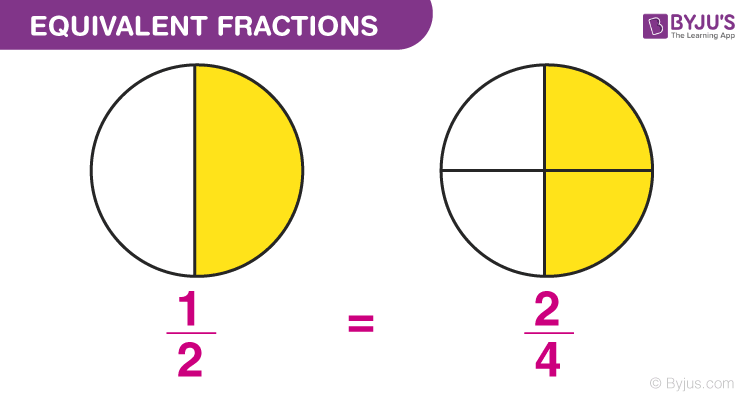# Equivalent Fractions

Equivalent fractions are the fractions that have different numerator and denominator but are equal to the same value. For example, 2/4 and 3/6 both are equal to ½. Hence they are equivalent in nature. These fractions represent the same proportion of the whole. Also learn Like Fractions Unlike Fractions, here.

For each fraction, we can find its equivalent fraction by multiplying both numerator and denominator with the same number. For example, we have to find the third equivalent fraction of ⅔; then we have to multiply ⅔ by 3/3. Hence,

⅔ x (3/3) = 6/9

## Equivalent Fractions Definition

Equivalent fractions state that two or more than two fractions are said to be equal if both results are the same fraction after simplification. Let us say, a/b and c/d are two fractions, after the simplification of these fractions, both result in equivalent fraction, say e/f, then they are equal to each other.

For example, the equivalent fraction of 1/3 is 5/15, because if we simplify 5/15, then the resulted fraction is the same.The biggest question here can be, why do they have equal values in spite of having different number?

The answer to this question is that, as the numerator and denominator are not co-prime numbers, therefore they have a common multiple which on division given an exactly the same value.

Take for an example:

1/2 = 2/4 = 4/8

But, it is clearly seen that the above fractions have different numerators and denominators.

Dividing both numerator and denominator by their common factor, we have:

$\large \frac{4 \div 4}{8 \div 4}$

=1/2

In the same way, if we simplify 2/4, again get 1/2.

$\large \frac{2\div 2}{4\div 2}$

= 1/2

## How to Find Equivalent Fractions

These fractions are actually the same because when we multiply or divide both the numerator and the denominator by the same number, the value of the fraction actually doesn’t change. Therefore, equivalent fractions, when reduced to their simplified value, will all be the same.

For example, consider the fraction 1/5

Multiplying numerator and denominator with 2, we get 1/5 = 2/10

Multiplying numerator and denominator with 3, we get 1/5 = 3/15

Multiplying numerator and denominator with 4, we get 1/5 = 4/20

Therefore, we can conclude that,

1/5 = 2/10 = 3/15 = 4/20

Keep in mind that we can only multiply or divide by the same numbers to get an equivalent fraction, and not addition or subtraction. Simplification to get equivalent numbers can be done till a point where both the numerator and denominator should still be whole numbers.

Try the following question to check your understanding of the concept of equivalent fractions.

## Equivalent Fractions Chart

 Unit Fraction Equivalent Fractions 1/2 2/4, 3/6, 4/8.. 1/3 2/6, 3/9, 4/12.. 1/4 2/8, 3/12, 4/16.. 1/5 2/10, 3/15, 4/20,.. 1/6 2/12, 3/18, 4/24,.. 1/7 2/14, 3/21, 4/28,.. 1/8 2/16, 3/24, 4/32,.. 1/9 2/18, 3/27, 4/36,..

Example 1: The given fractions 5/16 and x/12 are equivalent fractions, then find the value of x.

Solution:

Given: 5/16 = x/12

x = (5 x 12)/16

x = 60/16

x =15/4

Therefore, the value of x is 15/4.

Example 2: Two fractions 3/5 and 4/x are equivalent. Find the value of x.

Solution: Given,

3/5 = 4/x

x = (4 x 5)/3

x = 20/3

Example 3: What fractions are same as ¼?

Solution: To find equivalent fractions of ¼ we need to multiply the numerator and denominator by same numbers. Hence,

¼ x (2/2) = 2/8

¼ x (3/3) = 3/12

¼ x (4/4) = 4/16

¼ x (5/5) = 5/20

And so on.

Example 4: What is the fourth equivalent fraction of ¾?

Answer: To find the fourth equivalent fraction of ¾, we need to multiply numerator and denominator by 4. Hence,

¾ x (4/4) = 12/16

Have persisting doubts on any topic? Try reaching out to our mentors only with BYJU’S – The Learning App.

### Practice Questions

1. Find the equivalent fraction of 8/10
2. Write the fraction three-sevenths as an equivalent fraction with a denominator of 21.
3. Write the fraction five-eighth as an equivalent fraction with a denominator of 24.

## Frequently Asked Questions – FAQs

### What are equivalent fractions?

When two or more fractions which have different numerator and denominator but results in same value after simplification are said to be equivalent fractions.

### What are the examples of equivalent fractions?

The examples of equivalent fractions are:
2/4 and 3/6
⅓ and 3/9
⅕ and 5/25
¾ and 12/16

### How to determine whether fractions are equivalent?

We need to simplify the given fractions to find whether they are equivalent or not. Suppose 3/9 and ⅔ are two fractions. 3/9 can be further simplified to ⅓, but ⅓ is not equal to ⅔. Therefore they are not equivalent.
But, ⅚ and 10/12 are equivalent fractions because of 10/12 = ⅚.

### What is the equivalent fraction of ⅗?

To find the equivalent fraction of ⅗, we need to multiply both numerator and denominator by the same number. Hence,
⅗ x (2/2) = 6/10

### What are the equivalent fractions of 5/10?

5/10 is equal to ½ after simplification. Hence the equivalent fractions of 5/10 are:
½, 2/4, 3/6, 4/8 and so on.

Amazing apps study

2. Jas Jas

Hi, yes please. Can you do it for 8/9?

1. lavanya

The equivalent fraction to 8/9 is 16/18

1. how did you find it please teach me?

2. lavanya

Multiply numerator and denominator by a common factor, to get the equivalent fractions.
(8/9) x (2/2)
(8×2)/(9×2)
16/18